# Excel Charts - Surface Chart

Surface charts are useful when you want to find the optimum combinations between two sets of data. As in a topographic map, the colors and patterns indicate the areas that are in the same range of values.

To create a Surface chart, ensure that both the categories and the data series are numeric values.

Step 1 − Arrange the data in columns or rows on the worksheet.

Step 2 − Select the data.

Step 3 − On the INSERT tab, in the Charts group, click the Stock, Surface or Radar Chart icon on the Ribbon.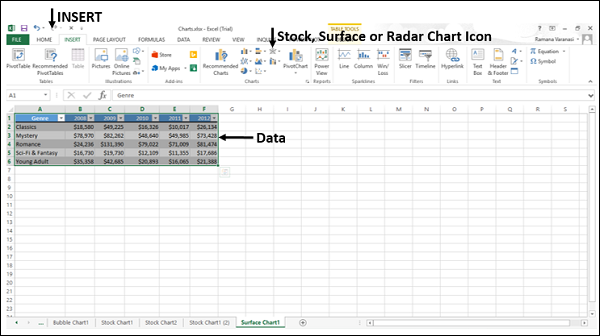You will see the different types of available Surface charts.

A Surface chart has the following sub-types −

• 3-D Surface
• Wireframe 3-D Surface
• Contour
• Wireframe Contour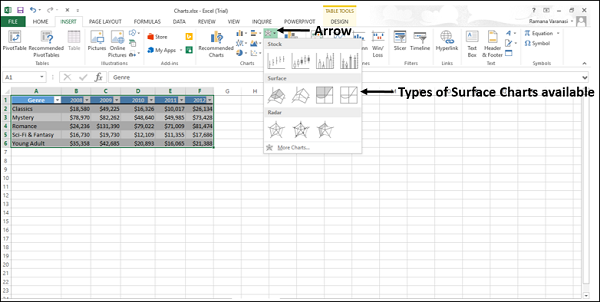Step 4 − Point your mouse on each of the icons. A preview of that chart type will be shown on the worksheet.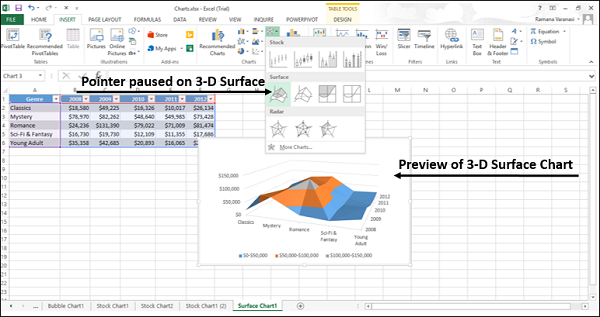Step 5 − Double-click the chart type that suits your data.

In this chapter, you will understand when each of the Surface chart types is useful.

## 3-D Surface

3-D Surface chart shows a 3-D view of the data, which can be imagined as a rubber sheet stretched over a 3-D Column chart. It is typically used to show relationships between large amounts of data that may otherwise be difficult to see.

Color bands in a Surface chart −

• Do not represent the data series

• Indicate the difference between the values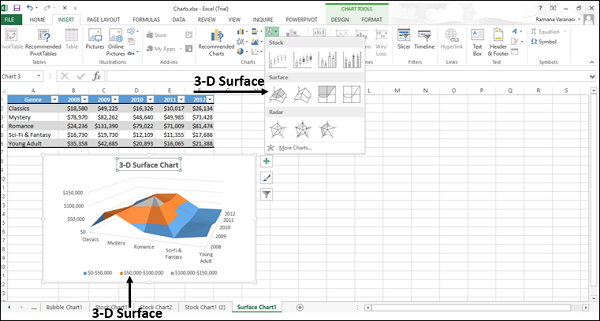You can use a 3-D Surface chart −

• When the categories and the series are both numeric values.

• To show the trends in values across two dimensions in a continuous curve.

## Wireframe 3-D Surface

A Wireframe 3-D Surface chart is a 3-D Surface chart shown without color on the surface. This chart shows only the lines. A Wireframe 3-D Surface chart is not easy to read, but it can plot large data sets much faster than a 3-D Surface chart.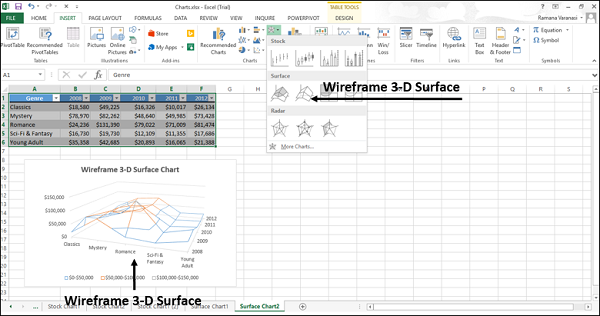You can use a Wireframe 3-D Surface chart −

• To show the trends in values across two dimensions in a continuous curve.

• When the categories and the series are both numeric values.

• When the data curves behind itself.

## Contour

Contour charts are Surface charts viewed from above, similar to the 2-D topographic maps.

In a Contour chart,

• The color bands represent specific ranges of the values.

• The lines connect the interpolated points of equal value.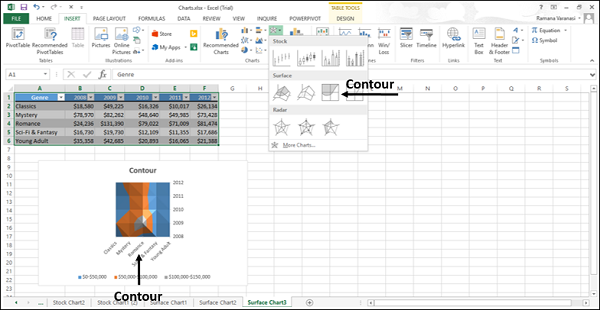Use Contour chart −

• To show the 2-D top view of a 3-D surface chart.

• To represent the ranges of the values using color.

• When both the categories and the series are numeric.

## Wireframe Contour

Wireframe Contour charts are also Surface charts viewed from above. A Wireframe chart shows only the lines without the color bands on the surface. Wireframe Contour charts are not easy to read. You can use a 3-D Surface chart instead.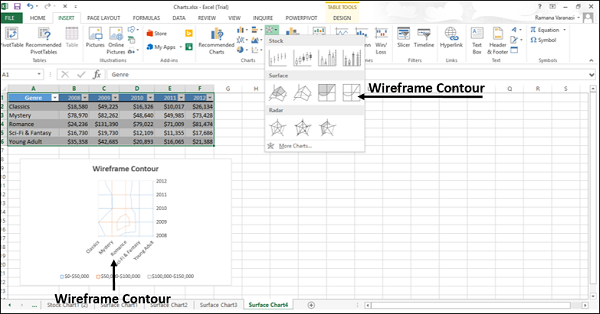Use Wireframe Contour chart

• To show the 2-D top view of a 3-D Surface chart only with lines.

• Both the categories and the series are numeric.

Consider using a Contour chart instead, because the colors add detail to this chart type.